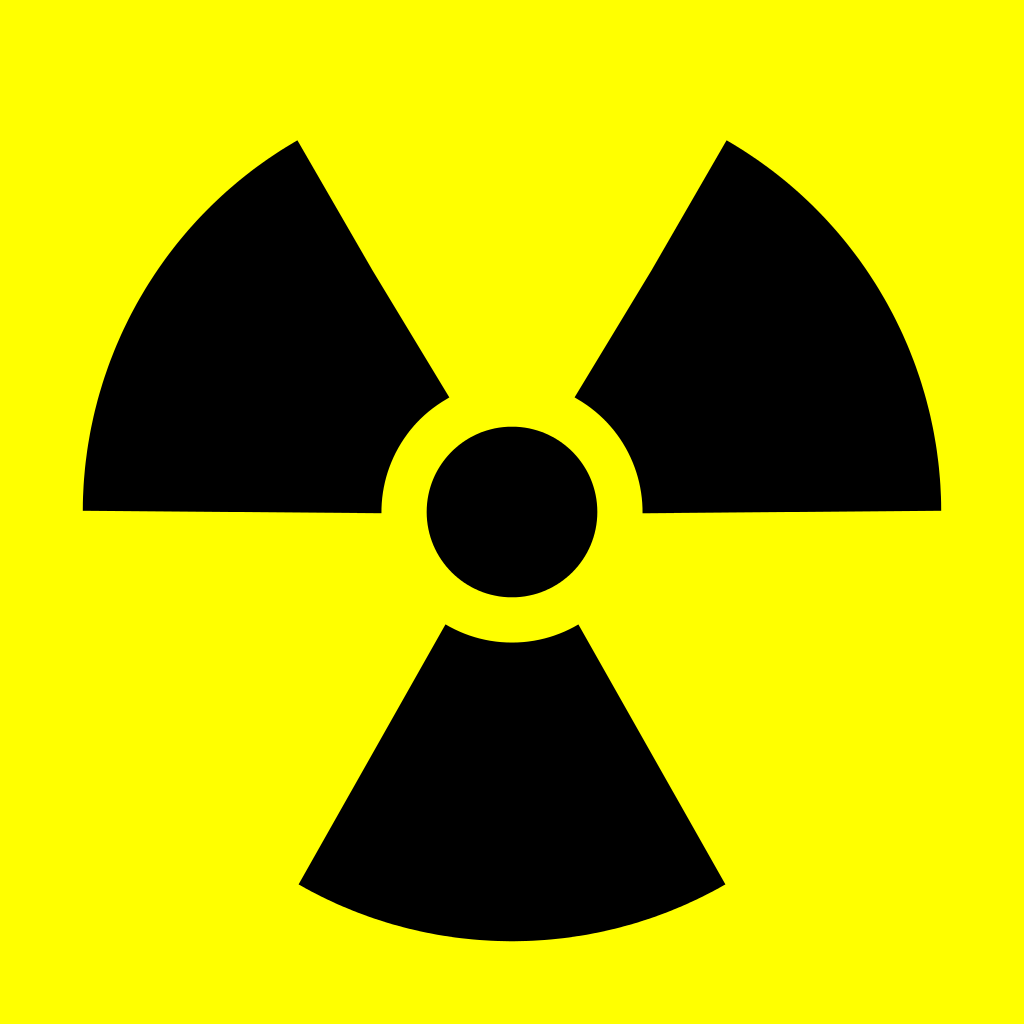The symbol is made from three concentric circles and three equally spaced diameters of the large circle. The diameter of the large circle is $12$, the diameter of small circle is $4$ and the diameter of smallest circle is $2$. What is the area of the symbol (shaded region) ?